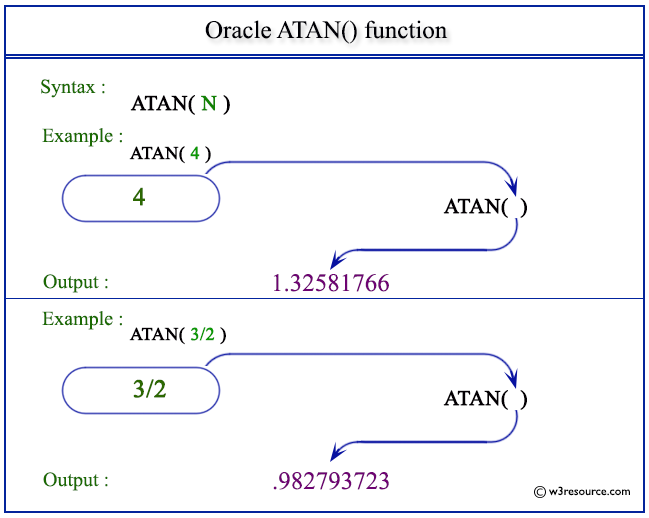# Oracle ATAN() function

## Description

The ATAN() function is used to calculate the angle value (in radians) of a specified tangent. The specified number can be in an unbounded range and returns a value in the range of -pi/2 to pi/2, expressed in radians.
The function takes any numeric or nonnumeric data type (can be implicitly converted to a numeric data type) as an argument.
If the argument is BINARY_FLOAT, then the function returns BINARY_DOUBLE. Otherwise, the function returns the same numeric data type as the argument.

Syntax:

`ATAN(N)`

Parameters:

Name Description
N A number whose arc tangent value is to be retrieved.

Pictorial Presentation of ATAN() functionExample:

The statement below will return the arc tangent value of the number specified as an argument.

``````SELECT ATAN(4) FROM dual;
```
```

Here is the result.

```   ATAN(4)
----------
1.32581766
```

Example: ATAN() function using negative value

This statement below will return the arc tangent value of the number defined as an argument.

```SELECT ATAN(-4) FROM dual;
```

Here is the result.

```  ATAN(-4)
----------
-1.3258177
```

Example: ATAN() function using a division

This statement below will return the arc tangent value of the number (3 divided by 2) defined as arguments.

``````SELECT ATAN(3/2) FROM dual;
```
```

Here is the result.

``` ATAN(3/2)
----------
.982793723
```

Previous: ASIN
Next: ATAN2

﻿